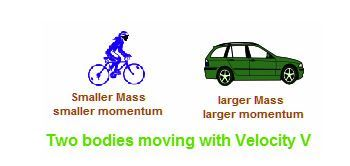# Momentum Formula

According to newton’s law of motion, all moving bodies continue to be in the state of rest or motion unless interfered by some external force. The same principle can be applied to momentum i.e, if the mass and velocity of an object remain the same, then the momentum of the object remains constant.

Momentum is associated with the mass of the moving body and can be defined as the quantity of motion measured as a product of mass and velocity.The momentum formula is given by,

p=mv

Where,

p is the momentum of the body,

m is the mass of the body and

v is the velocity of the body.

The S.I unit for momentum is Kgms-1.

Example 1

A car of mass 600 Kg is moving with a velocity of 10 m/s. Determine its Momentum.

Solution:

Given:

Mass, m = 600 Kg,

Velocity, v = 10 m/s

Momentum is given as

p = mv

= 600 × 10

= 6000 Kgms-1.

Example 2

A bike is moving at the rate of 30 m/s carrying a momentum of 5000 kgm/s. Determine its mass.

Solution:

Given:

Velocity v = 30m/s,

Momentum p = 5000 kgm/s,

Momentum is given as

p = m × v

Mass, m = p / v

= 5000 / 30

m = 166.66 Kg